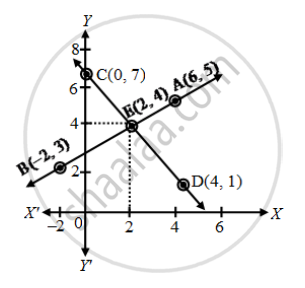# Show graphically that the system of equations x – 4y + 14 = 0; 3x + 2y – 14 = 0 is consistent with unique solution. - Mathematics

Sum

Show graphically that the system of equations x – 4y + 14 = 0; 3x + 2y – 14 = 0 is consistent with unique solution.

#### Solution

The given system of equations is

x – 4y + 14 = 0 ….(1)

3x + 2y – 14 = 0 ….(2)

x – 4y + 14 = 0 ⇒ y = \frac { x + 14 }{ 4 }

 x 6 -2 y 5 3 Points A B

3x + 2y – 14 = 0 ⇒ y = \frac { -3x + 14 }{ 2 }

 x 0 4 y 7 1 Points C DThe given equations representing two lines, intersect each other at a unique point (2, 4). Hence, the equations are consistent with unique solution.

Concept: Graphical Method of Solution of a Pair of Linear Equations
Is there an error in this question or solution?